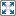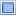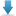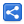# Java - Synchronized Blocks

[Last Updated: Apr 1, 2016]

Other than using synchronized methods, code can be synchronized within a block surrounded by curly brackes `{ }`.

For example:

```  private synchronized void updateData () {
//other code here
}
```

can be rewritten as

```  private void updateData () {
synchronized(this){
//other code here
}
}```

Using `synchronized(this){}` we are saying that the block should be locked on 'this' instance.

Technically, there's no difference between the above two but with synchronized block we can have more control with regard to the scope of intrinsic lock.

By limiting the scope of synchronization we can gain some performance improvements too. Synchronization is a costly process in terms of performance. It's good to synchronize smaller blocks of code instead of a complete method.

We can use any object instance in the synchronized block: `synchronized(anyInstance){}`
That means synchronized blocks have the flexibility of using other objects as locks whereas a synchronized method would lock only on the enclosing object instance. 'anyInstance' in above snippet cannot be null otherwise NullPointerException will be thrown.

In following examples we will explore the benefits of using 'synchronized blocks' over 'synchronized methods'.

## Reducing scope of lock for performance improvements

Consider a commonly used lazy initialization of an object in multithreaded environment:

```public class LazyInitBlockDemo {
private List<String> list;

public static void main (String[] args) throws InterruptedException {
LazyInitBlockDemo obj = new LazyInitBlockDemo();

});
});

}

private List<String> getList () {
if (list == null) {
list = new ArrayList<>();
}
return list;
}
}```

Output:

Above code is not thread safe. Running multiple time could occasionally give the different hashCode of the list, meaning it is initialized twice by interfering threads:

```thread2 : 999575674

To fix that we must synchronize the initializing code:

``` private synchronized List<String> getList () {
if (list == null) {
list = new ArrayList<>();
}
return list;
}```

This will fix the issue but for performance reason, it is not good to synchronize the getter all the time because the list ,after all, is initialized only once. Let's use synchronized block conditionally:

```    private volatile List<String> list;
......
private List<String> getList () {
if (list == null) {
synchronized(this) {
if(list == null) {
list = new ArrayList<>();
}
}
}
return list;
}```

Above pattern is also known as Double-checked locking. It's used in singleton pattern as well.

The second null checking is referred as 'Double-checking' the variable. If list has already been accessed by another competing thread, it may have already been initialized. If so, return the initialized variable.

Declaring our list as volatile ensures that the read must happen after the write has taken place, and the reading thread will see the correct value of list instance.

## Using different lock objects

With synchronized block we can use any arbitrary object as lock. Let's lazy initialize multiple objects from our previous example:

```public class MultipleLocksDemo {
private volatile List<String> list1;
private volatile List<String> list2;
private final Object lock1 = new Object();
private final Object lock2 = new Object();

public static void main (String[] args) throws InterruptedException {
MultipleLocksDemo obj = new MultipleLocksDemo();

System.identityHashCode(obj.getList1()));
System.identityHashCode(obj.getList2()));
});
System.identityHashCode(obj.getList1()));
System.identityHashCode(obj.getList2()));
});

}

private List<String> getList1 () {
if (list1 == null) {
synchronized (lock1) {
if (list1 == null) {
list1 = new ArrayList<>();
}
}
}
return list1;
}

private List<String> getList2 () {
if (list2 == null) {
synchronized (lock2) {
if (list2 == null) {
list2 = new ArrayList<>();
}
}
}
return list2;
}
}```

Output:

```thread1 list1 : 571142194
```

Generally speaking if we are accessing multiple shared resources in different thread, each resource access should be locked on different objects. We can use the shared resource instance as lock itself given that it's not null and there's a single resource access in the synchronized block.

```public class MultipleLocksDemo2 {
private List<String> list1 = new ArrayList<>();
private List<String> list2 = new ArrayList<>();

public static void main (String[] args) {
MultipleLocksDemo2 obj = new MultipleLocksDemo2();

for (int i = 0; i < 5; i++) {
obj.printLists();
}
});
for (int i = 0; i < 5; i++) {
obj.printLists();
}
});

}

public void addToList1 (String s) {
synchronized (list1) {
}
}

public void addToList2 (String s) {
synchronized (list2) {
}
}

public void printLists () {
.getName();

synchronized (list1) {
list1.stream()
.forEach(l -> System.out.println(name + " : " + l));
}
synchronized (list2) {
list2.stream()
.forEach(l -> System.out.println(name + " : " + l));
}
}
}```

In above example we need synchronization on add and print methods. Removing synchronized block will cause `java.util.ConcurrentModificationException`

## Synchronized by String value

We can use String.intern as locks on strings values but that's not very reliable approach as discussed here.

In the following example we are using a map of locks for read/write operations on the file objects.

Generally speaking we should always use objects locks which our code maintains instead of relying on JVM managed objects.

```public class SyncBlockStringLock {
private Map<String, Object> locks = new HashMap<>();

private static final File rootFolder = new File("d:\\test");

static {
if (!rootFolder.exists()) {
rootFolder.mkdir();
}
}

public static void main (String[] args) {
SyncBlockStringLock obj = new SyncBlockStringLock();
for (int i = 0; i < 5; i++) {
String path = rootFolder.getAbsolutePath() + File.separatorChar + i;
obj.writeData(path, " thread1 data " + i);
}
});

for (int i = 0; i < 5; i++) {
String path = rootFolder.getAbsolutePath() + File.separatorChar + i;
obj.writeData(path, " thread2 data " + i);
}
});

}

private void writeData (String path, String data) {
synchronized (getLock(path)) {
try {
Files.write(Paths.get(path), data.getBytes());
} catch (IOException e) {
e.printStackTrace();
}
}
}

private void readData (String path) {
synchronized (getLock(path)) {
String s = null;
try {
} catch (IOException e) {
e.printStackTrace();
}
System.out.println(s);
}
}

private Object getLock (String path) {
if (!locks.containsKey(path)) {
locks.put(path, new Object());
}

return locks.get(path);
}
}```

## Example Project

Dependencies and Technologies Used:

• JDK 1.8
• Maven 3.0.4

 Synchronized Blocks ExampleSelect AllDownloadShare